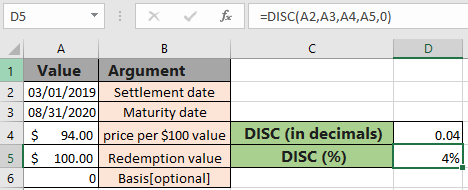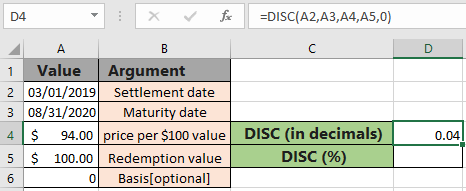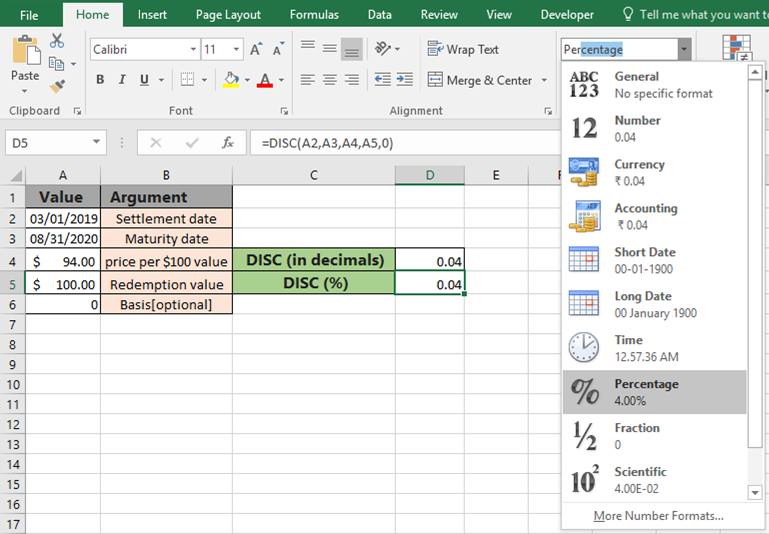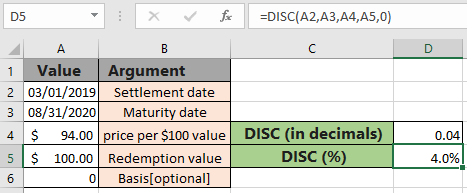# How to use the DISC function in ExcelIn this article, we will learn How to use the DISC function in Excel.

What is the Discount rate for a security?

Discount for a security is the interest used to convert between future value and present value. A future value can be "discounted" by a given interest rate to determine the present value. The discount rate is calculated using the mathematical formula shown below.

Discount rate = ( 1 - pr / redemption ) * ( B / DSM )

Here pr is the price per \$100 face value for the security. B is the number of days in a year, based on the selected basis method and DSM is the number of days between settlement and maturity. Learn how to calculate the discount rate using the DISC function in Excel.

DISC function in Excel

DISC function is a financial function which returns the discount rate as percentage using the terms for a bond or security. Below is detailed function syntax and arguments

DISC function syntax:

 =YIELD ( Settlement , Maturity, pr, redemption, [basis])

settlement - Settlement date of security

Maturity - maturity date of security

pr - Price per \$ 100 face value

redemption - redemption value

basis - [optional] Day count basis. Default is 0 and for see  the table below.

 Basis Day count basis 0 US (NASD) 30 / 360 1 actual / actual 2 actual / 360 3 actual / 365 4 European 30 / 360

Example :

All of these might be confusing to understand. Let's understand this function using an example. Here we have some terms for the security stated as per required arguments and we need to calculate the discount rate for the security.

Use the formula:

 =DISC(A2,A3,A4,A5,0)

Day count basis selected as US (NASD) 30 / 360The function returns 0.04 as result, which means 4% in Excel. Change the format of the cell from General to Percentage to get the value in Percentage. Select the percentage from the format cell drop down list as shown below.Selecting the Percentage, the 0.04 turns to 4% in cell as shown below.As you can see, the same formula returns the same discount rate in percentage format. Learn more about the bond valuation formulas and related functions here at Exceltip.

Here are some observational notes using the DISC function shown below.
Notes :

1. The function returns the Discount rate in decimal (0.12) value or percent (12%) value. Both are considered the same in Excel.
2. Excel stores dates as serial numbers and is used in calculation by the function. So it’s recommended to use dates as cell reference instead of giving direct argument to the function. Check the date values if the function returns #VALUE! Error.
3. The security settlement date is the date after the issue date when the security is traded to the buyer.
4. Arguments like settlement date, maturity date & basis are truncated to integers.
5. The function returns the #VALUE! Error if the settlement date & maturity date is not a valid date format.
6. The function returns the #NUM! Error if:
1. If the basis argument is either < 0 or  > 4.
2. If maturity date settlement value.
3. If pr argument ? 0
4. if redemption argument is  < 0

Hope this article about How to use the DISC function in Excel is explanatory. Find more articles on financial formulas here. If you liked our blogs, share it with your friends on Facebook. And also you can follow us on Twitter and Facebook. We would love to hear from you, do let us know how we can improve, complement or innovate our work and make it better for you. Write to us at info@exceltip.com.

Related Articles

How to use the MIRR function in excel : returns the Modified interest rate of return for the financial data having Investment, finance rate & reinvestment_rate using the MIRR function in Excel.

How to use the XIRR function in excel : returns the Interest rate of return for irregular interval using the XIRR function in Excel

Excel PV vs FV function : find Present Value using PV function and future value using FV function in Excel.

How to use the RECEIVED function in excel : calculates the amount which is received at maturity for a bond with an initial investment (security) and a discount rate, there are no periodic interest payments using the RECEIVED function in excel.

How to use the NPER function in excel : NPER function to calculate periods on payments in Excel.

How to use the PRICE function in excel : returns the price per \$100 face value of a security that pays periodic interest using the PRICE function in Excel.

Popular Articles :

How to use the VLOOKUP Function in Excel : This is one of the most used and popular functions of excel that is used to lookup value from different ranges and sheets.

How to use the IF Function in Excel : The IF statement in Excel checks the condition and returns a specific value if the condition is TRUE or returns another specific value if FALSE.

How to use SUMIF Function in Excel : This is another dashboard essential function. This helps you sum up values on specific conditions.

How to use the COUNTIF Function in Excel : Count values with conditions using this amazing function. You don't need to filter your data to count specific values. Countif function is essential to prepare your dashboard.

Terms and Conditions of use

The applications/code on this site are distributed as is and without warranties or liability. In no event shall the owner of the copyrights, or the authors of the applications/code be liable for any loss of profit, any problems or any damage resulting from the use or evaluation of the applications/code.